# DAV Class 8 Maths Chapter 16 Brain Teasers Solutions

The DAV Class 8 Maths Solution and DAV Class 8 Maths Chapter 16 Brain Teasers Solutions of Rotational Symmetry offer comprehensive answers to textbook questions.

## DAV Class 8 Maths Ch 16 Brain Teasers Solutions

Question 1A.
Tick (✓) the correct option.

(i) How does the shape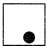look like after it rotates 180°?
(a)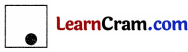(b)(c)(d)Solution:
(b)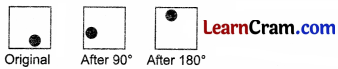Thus, option (b) is the correct answer.(ii) Letter ‘T’ has rotational symmetry of order
(a) 1
(b) 2
(c) 3
(d) 4
Solution:
(b) 2
The given letter has rotational symmetry of order 2.
Thus, option (b) is the correct answer.

(iii) The angle of rotation of a figure is 36° so, its order of rotational symmetry is
(a) 1
(b) 9
(c) 10
(d) 1
Solution:
(c) 10
Order of Rotational symmetry = $$\frac{360^{\circ}}{\text { Angle of rotation }}$$
= $$\frac{360^{\circ}}{36^{\circ}}$$ = 10°
Thus, option (c) is the correct answer.

(iv) Regular pentagon has a rotational symmetry of order
(a) 2
(b) 4
(c) 5
(d) 3
Solution:
(c) 5
The regular pentagon has a rotational symmetry of order 5.
Thus, option (c) is the correct answer.

(v) The angle of rotation of the given figure is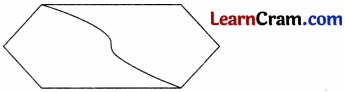(a) 90°
(b) 180°
(c) 270°
(d) 360°
Solution:
(b) 180°
The given figure has the rotational symmetry of order 2.
∴ Angle of rotation of figure = $$\frac{360^{\circ}}{2}$$ = 180°
Thus, the option (b) is the correct answer.Question 1B.

(i) Name two letters of English alphabet which have no rotational symmetry.
Solution:
Letters ‘F’ and ‘G’ of English alphabet have no rotational symmetry. (Answer may vary)

(ii) Find the angle and order of rotational symmetry of the figure given along side.Solution:
The given figure, repeats itself two times if it is rotated about a fixed point. So, the given figure has rotational symmetry of order 2.
Angle of rotation of the given figure = $$\frac{360^{\circ}}{2}$$ = 180°

(iii) Which letters of English alphabet have rotational symmetry of order 2?
Solution:
Letters ‘H’ and ‘S’ of English alphabet have rotational symmetry of order 2. (Answer may vary)

(iv) Does isosceles triangle exhibit rotational symmetry? Give reason.
Solution:
An isosceles triangle does not exhibit rotational symmetry as it does not repeat itself when it is rotated a full turn.

(v) Find the angle of rotation and order of rotation in the figure given along side.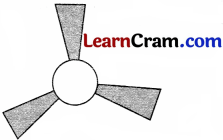Solution:
The given figure repeats itself three times when it is rotated about a fixed point. So, the given figure has the rotational symmetry of order 3.

Angle of rotation of the given figure = $$\frac{360^{\circ}}{3}$$ = 120°Question 2.
Name one figure each having rotational symmetry of order:
(i) 4
(ii) 2
(iii) 3
(iv) 6
Solution:
(i) Square has the rotational symmetry of order 4.The Angle of rotation of Square = $$\frac{360^{\circ}}{4}$$ = 90°

(ii) The rectangle has the rotational symmetry of order 2.Angle of rotation of rectangle = $$\frac{360^{\circ}}{2}$$ = 180°

(iii) Equilateral triangle has the rotational symmetry of order 3.Angle of rotation of equilateral triangle = $$\frac{360^{\circ}}{3}$$ = 120°

(iv) Regular hexagon has the rotational symmetry of order 6.Angle of rotation of regular hexagon = $$\frac{360^{\circ}}{6}$$ = 60°.Question 3.
In the word ‘MATHS’ which letter shows rotational symmetry of order 2?
Solution:
Only H shows the rotational symmetry.

Question 4.
List two letters of English alphabets which exhibit both line as well as rotational symmetry.
Solution:
H and O are the alphabet which shows both line and rotational symmetry.

Question 5.
Find the angle of rotation for each of the following.Solution:
(i) 180°
(ii) 90°
(iii) 72°
(iv) 90°

Question 6.
Write the order of rotational symmetry of a square and equilateral triangle with the help of rough sketches.
Solution:
(i)From the above figure, the order of symmetry of square is 4.

(ii)From the above figure, the order of symmetry of equilateral triangle is 3.### DAV Class 8 Maths Chapter 16 HOTS

Question 1.
Trace the figure below after it has been rotated 60° about the point G.Solution: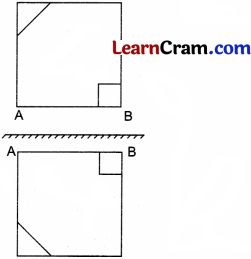Question 2.
Shade the triangles in the given figure in such a way that a shape is created with rotational symmetry of order 2.Solution:

###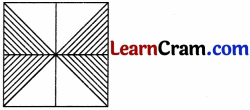### DAV Class 8 Maths Chapter 16 Enrichment Questions

Question 1.
‘Creating designs from transformations’
The word ‘transformation’ refers to a movement or change in geometric shape.
Reflection and rotation are the different kinds of transformations.

Produce patterns by performing the following transformation on the given figure.(i) Rotation of 90°, 180°, 270° about the vertex A.
Solution:(ii) A rotation of 90° about the vertex A followed by a reflection about the base of the figure so obtained.
Solution:Question 1.
Which of the following have rotational symmetry?Solution:
Figures (i), (iii) and (iv) have rotational symmetry about a fixed point.

Question 2.
Find the order of the rotational symmetry of the following shapes.
(a) Square
(b) Equilateral triangle
(c) Rectangle
Solution:
(а) The order of rotational symmetry of a square is 4.
(b) The order of rotational symmetry of equilateral triangle is 3.
(c) The order of rotational symmetry of a rectangle is 4.Question 3.
Draw the shapes of the figure whose rotational angles are

(a) 90°
Solution: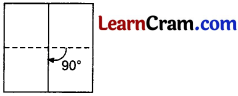Square has rotational angle of 90°.

(b) 120°
Solution:Equilateral triangle has the rotational angle 120°

(c) 180°
Solution: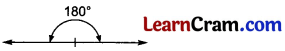Straight line has the rotational angle 180°

(d) 360°
Solution: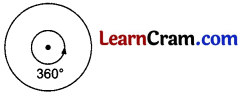Circle has the rotational angle 360°.Question 4.
Find the lines of symmetry of the following figures.
(a) Circle
Solution: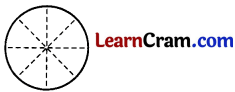Cricle has unlimited lines of symmetry.

(b) Square
Solution:Square has 4 lines of symmetry.

Question 5.
Find the number of lines of symmetry of the following figures.

(a)Solution:Here, the figure is a square which has 4 lines of symmetry.

(b)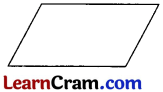Solution:Here, the figure is a parallelogram having no lines of symmetry.

(c)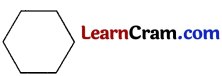Solution:Here, the figure is a regular hexagon which has 6 lines of symmetry.Question 6.
The order of rotation of a square is
(a) 4
(b) 2
(c) 1
(d) 3
Solution:
(a) is correct.

Question 7.
How many lines of symmetry a regular pentagon has?
(a) 6
(b) 5
(c) 7
(d) 8
Solution:
(b) is correct.

Question 3.
What is the order of rotation and angle of rotation for an equilateral triangle?
Solution:
Equilateral triangle has 3 order of rotation and it has angle of rotation 120°.

Multiple Choice Questions:

Question 1.
The lines of symmetry of a circle is
(a) 5
(b) 6
(c) 10
(d) Unlimited
Solution:
(d) Unlimited

Question 2.
The rotational angle of a square is
(a) 180°
(b) 90°
(c) 120°
(d) None of these
Solution:
(b) 90°

Question 3.
The lines of symmetry of a regular pentagon is
(a) 6
(b) 5
(c) 7
(d) 8
Solution:
(b) 5

Question 4.
The lines of symmetry of a regular hexagon is
(a) 4
(b) 6
(c) 8
(d) 12
Solution:
(b) 6

Question 5.
The angle of rotation of an equilateral triangle is
(a) 120°
(b) 100°
(c) 90°
(d) 180°
Solution:
(a) 120°